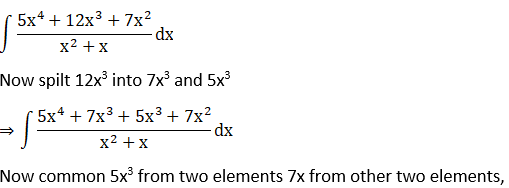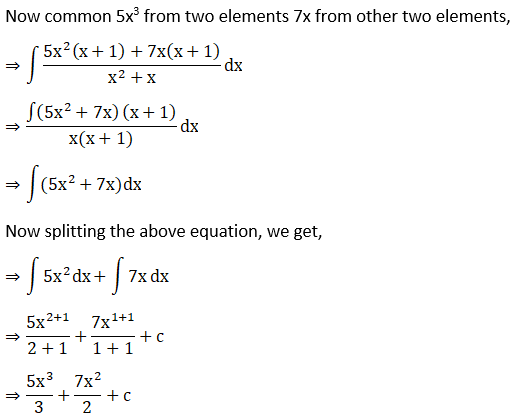# RD Sharma Solutions For Class 12 Maths Exercise 19.2 Chapter 19 Indefinite Integrals

The problems of the second exercise of this chapter are completely based on some standard results on integration. $\frac{d}{dx}\int \left ( f(x) \, dx \right ) = f (x)$ is the main formulae used while solving this exercise. This is created by our expert faculty in order to provide a clear understanding of important concepts by giving detailed explanations for the problems. This is a very useful tool for any student to get top marks in their Mathematics examinations. To know more about these topics, students can refer to RD Sharma Solutions for Class 12 Maths Chapter 19 Exercise 19.1 from the below provided links. Some of the crucial topics of this exercise are enlisted below.

• Some standard results on integration along with corollary
• Integration of trigonometric functions
• Integration of exponential functions
• Miscellaneous problems

## Download the PDF of RD Sharma Solutions For Class 12 Chapter 19 – Indefinite Integrals Exercise 19.2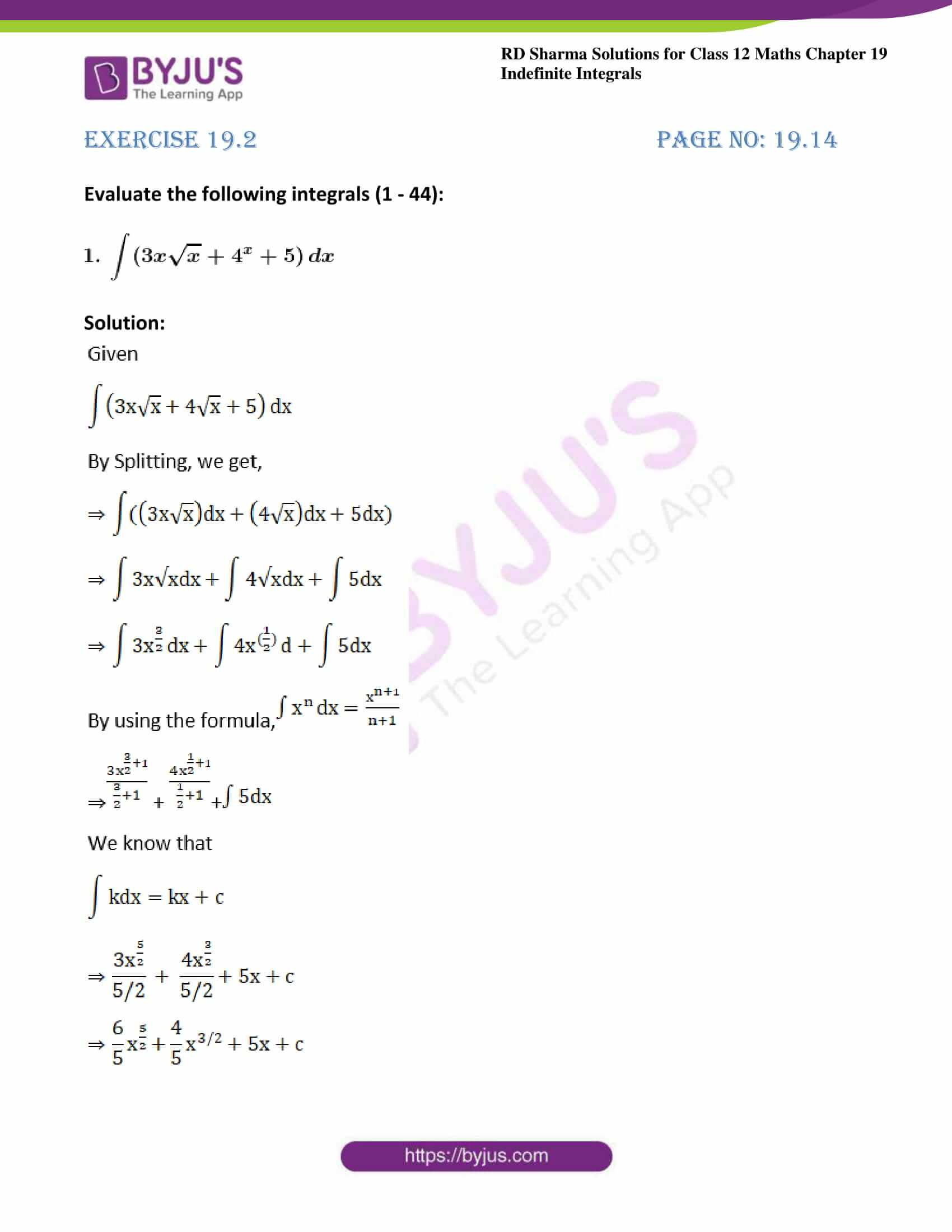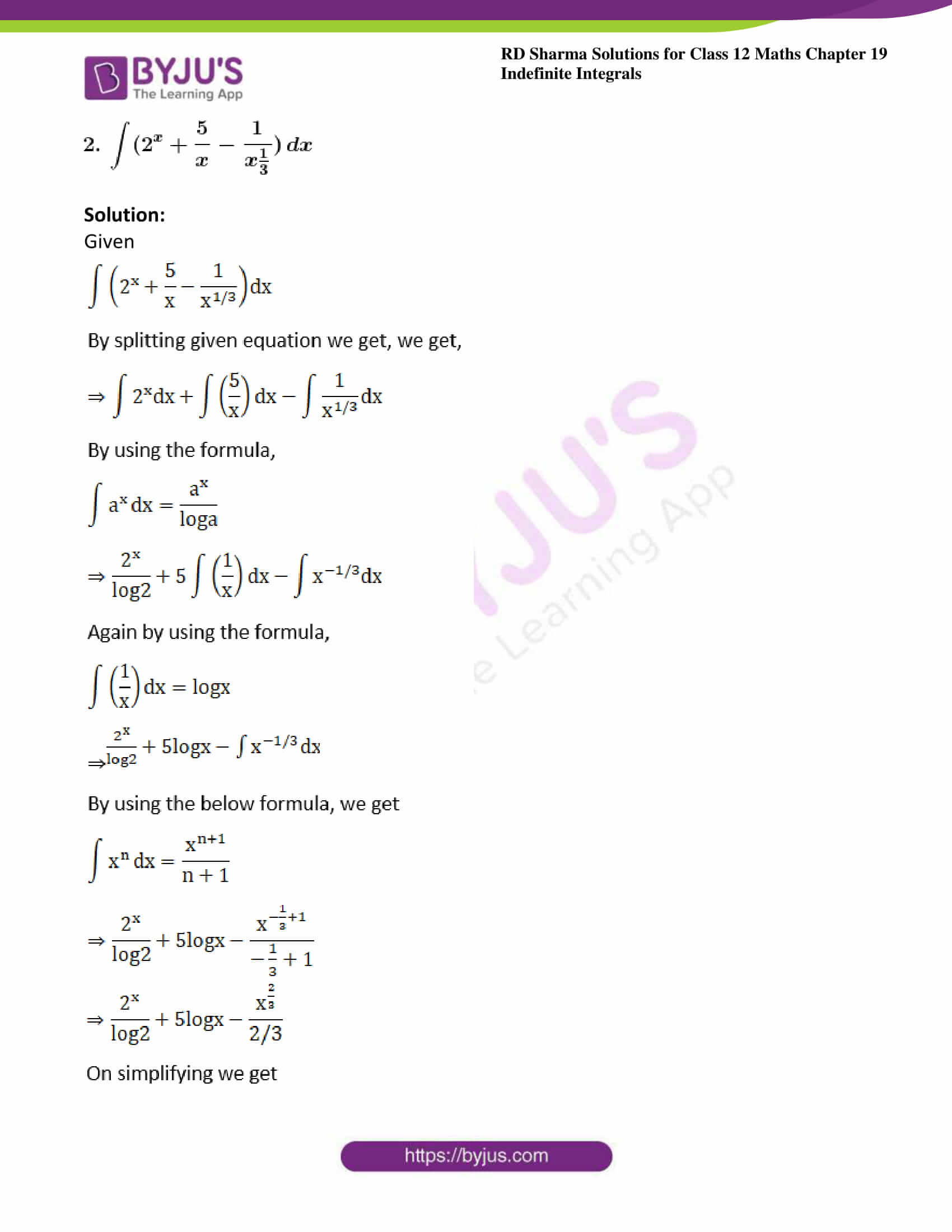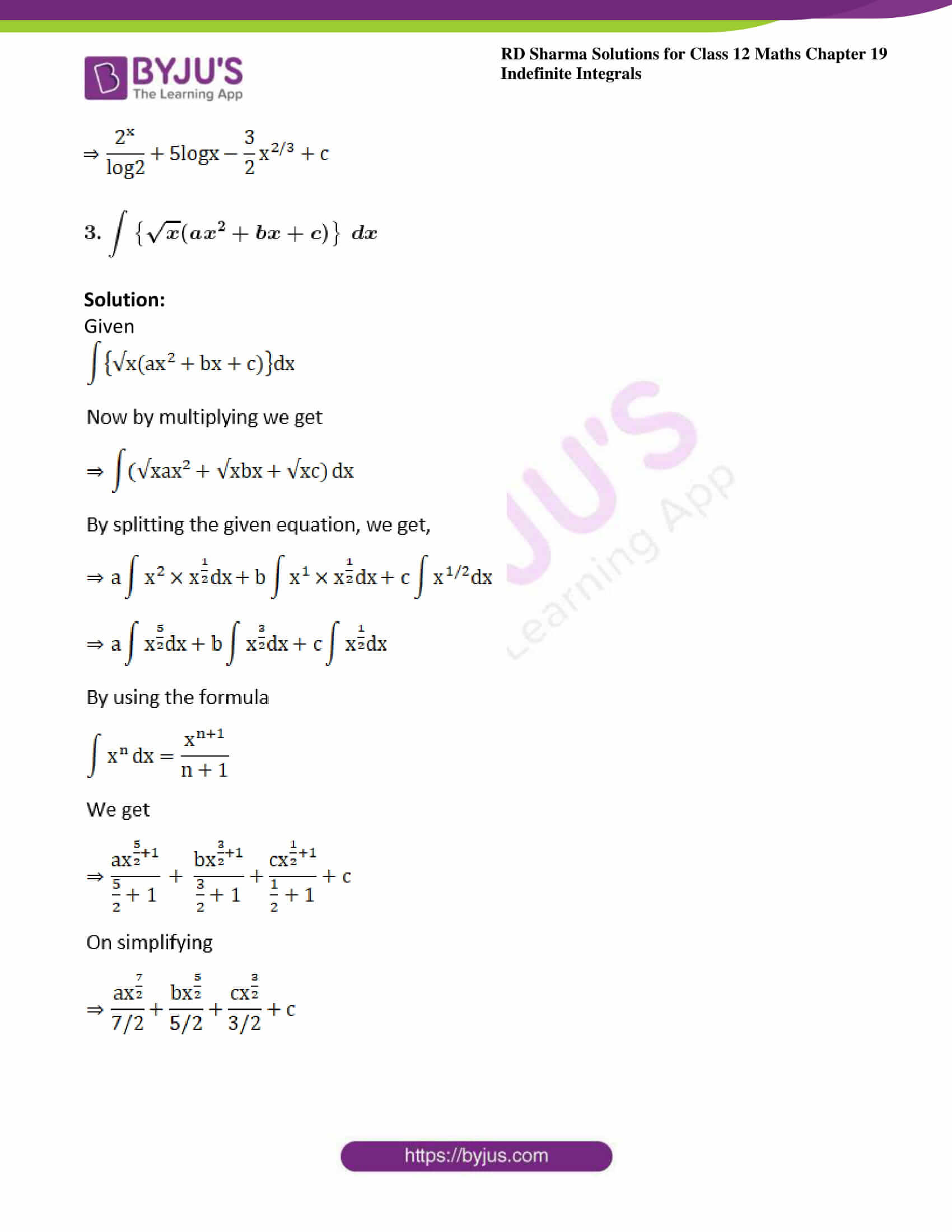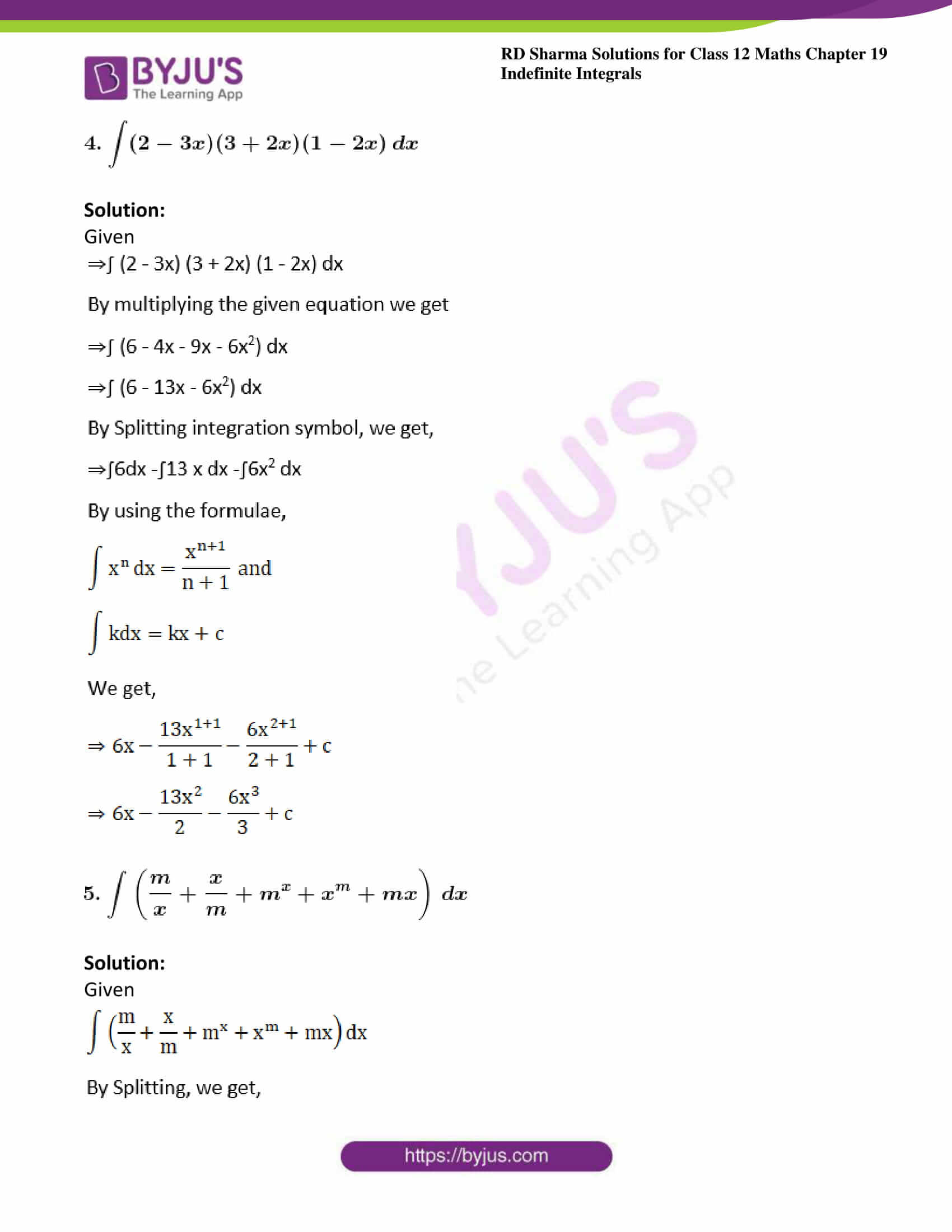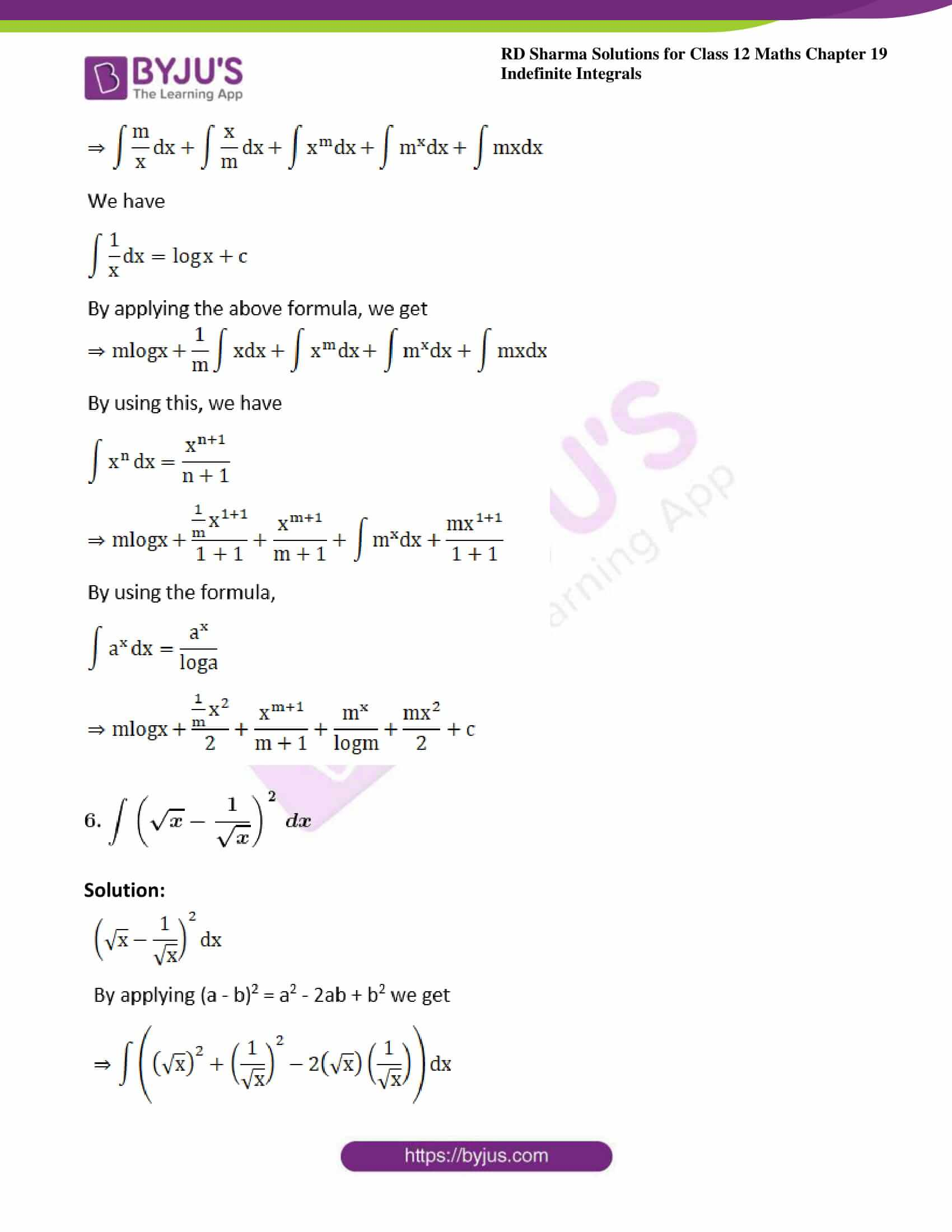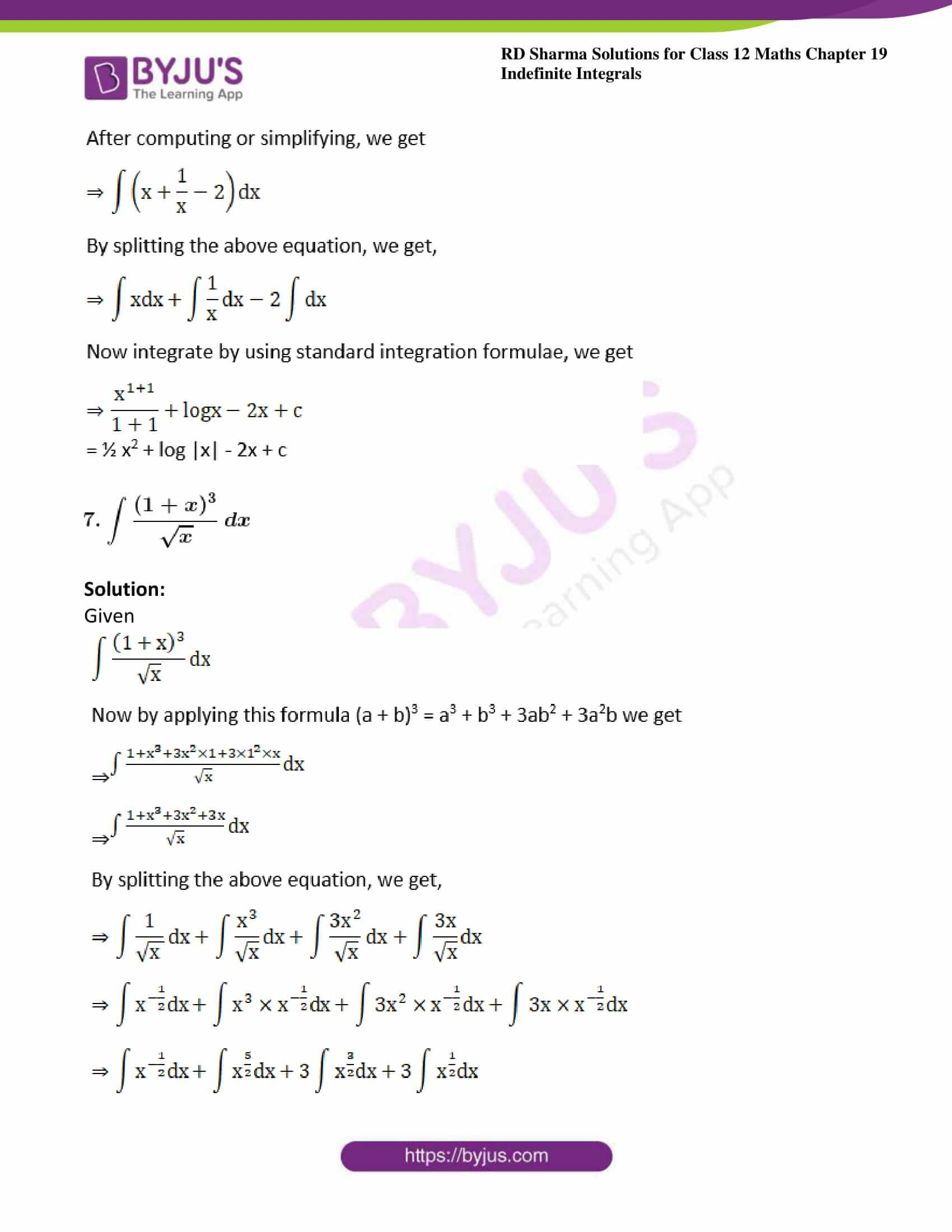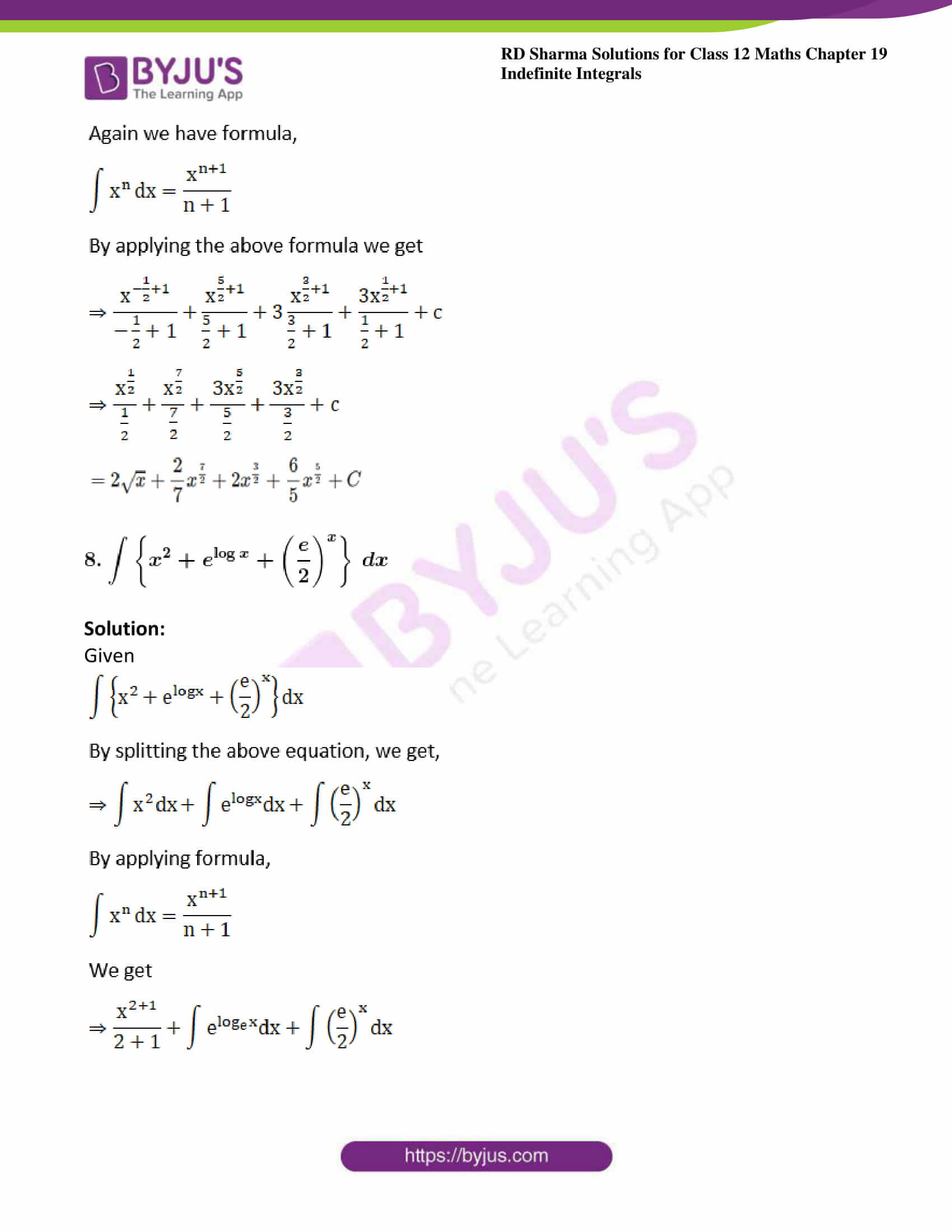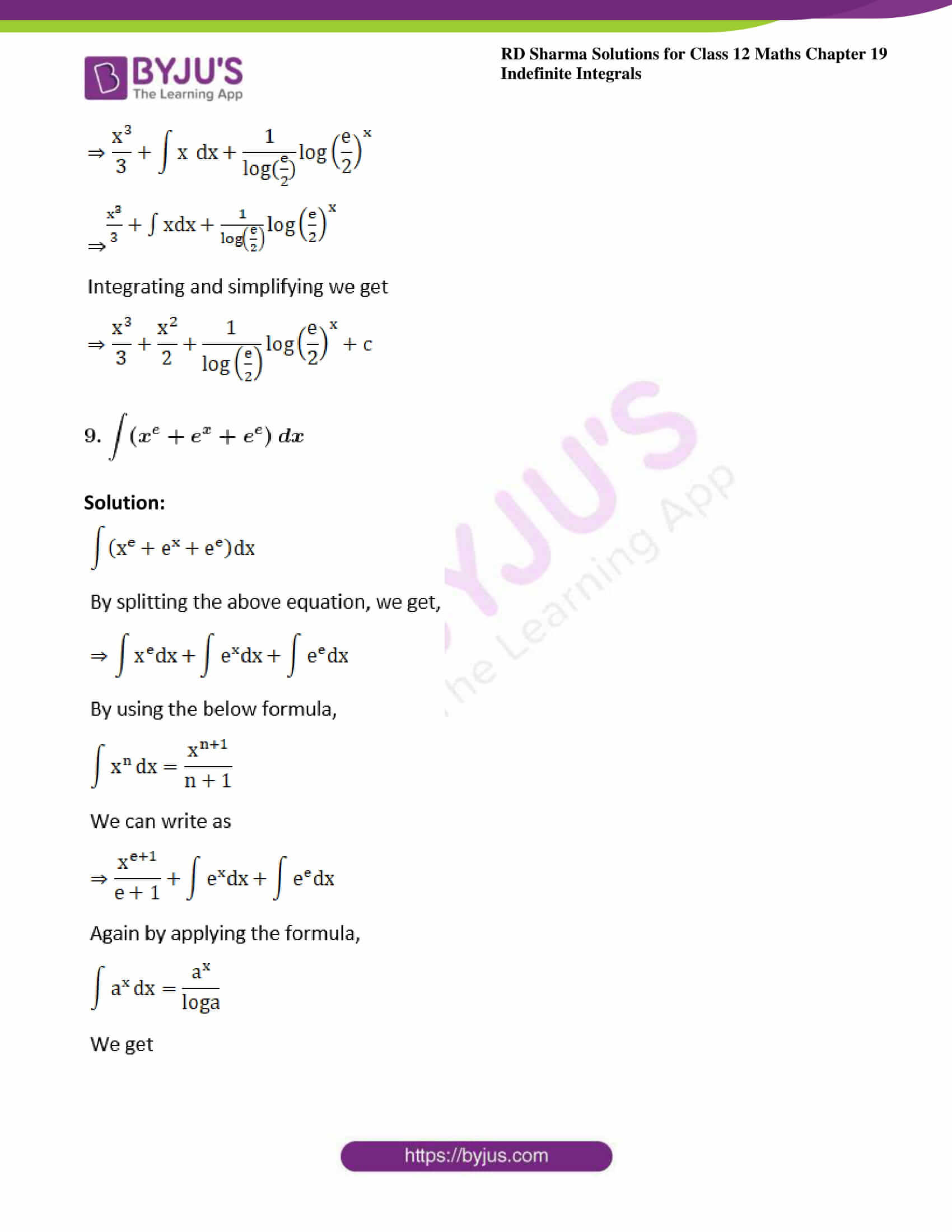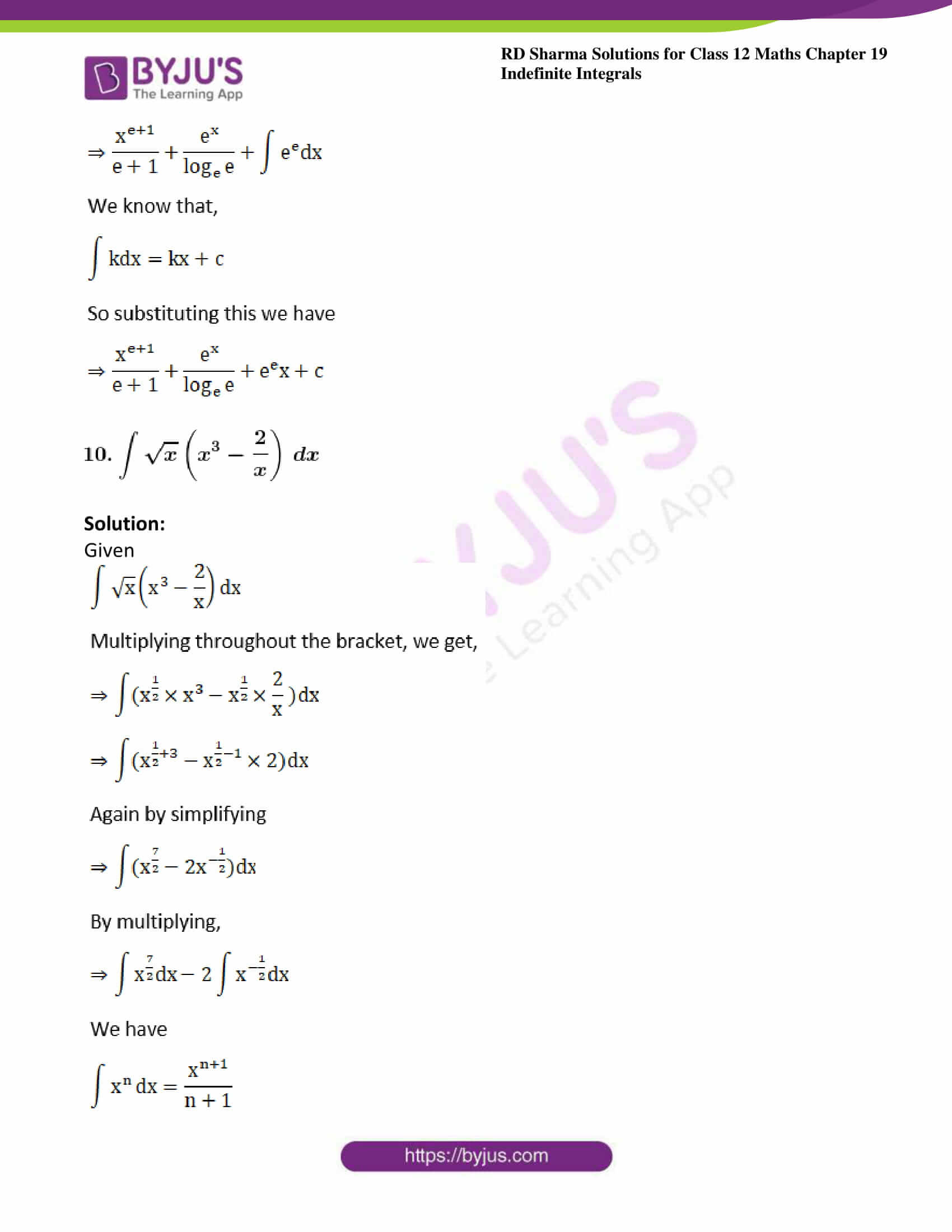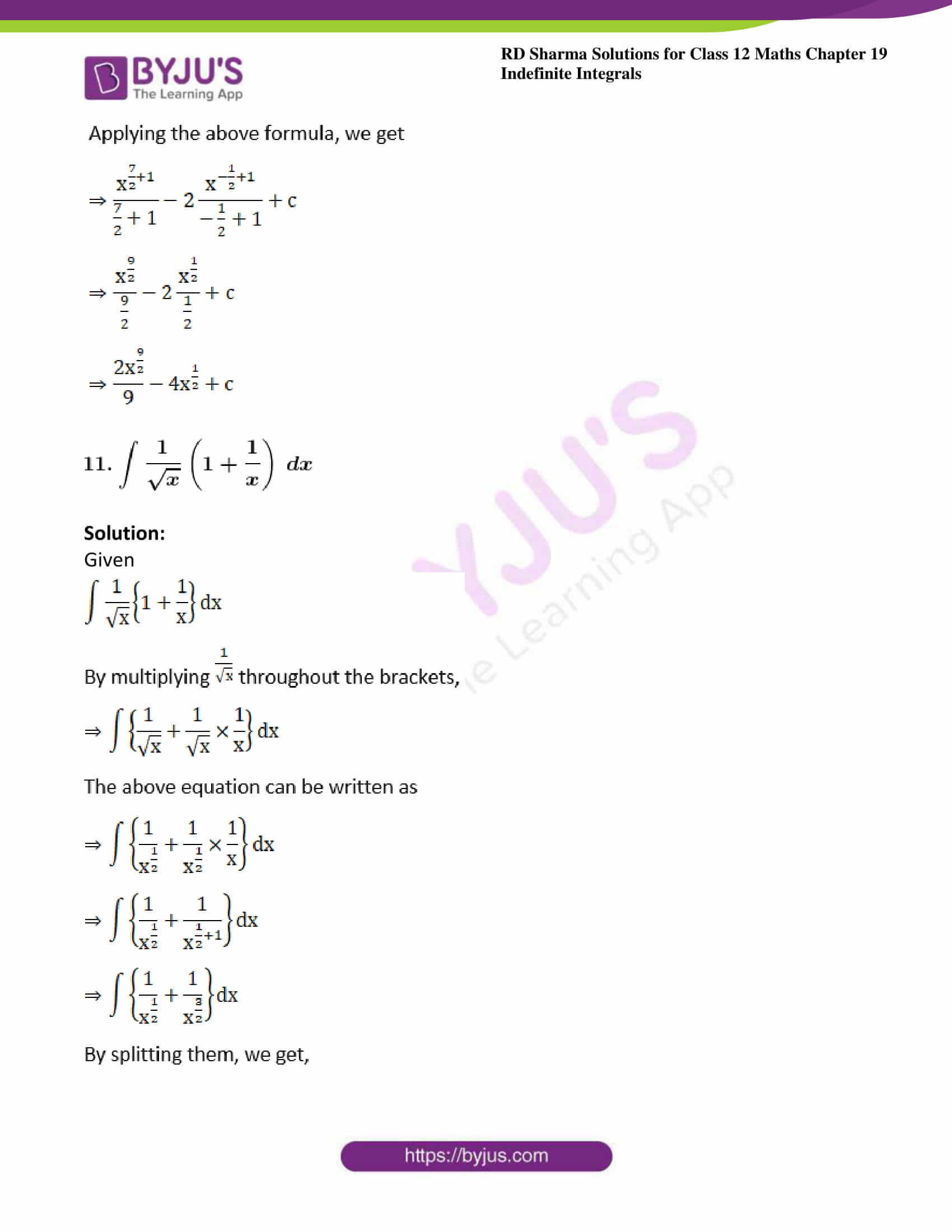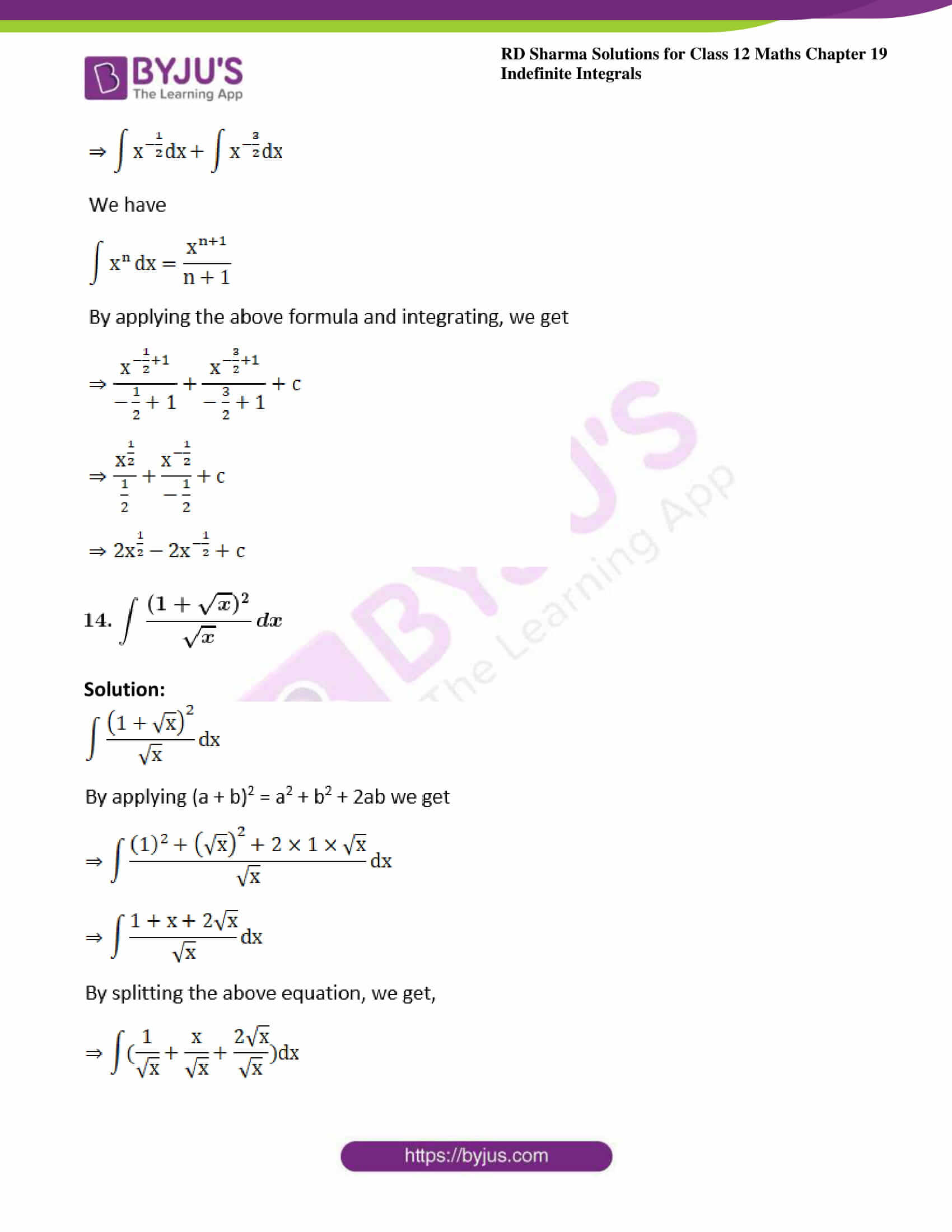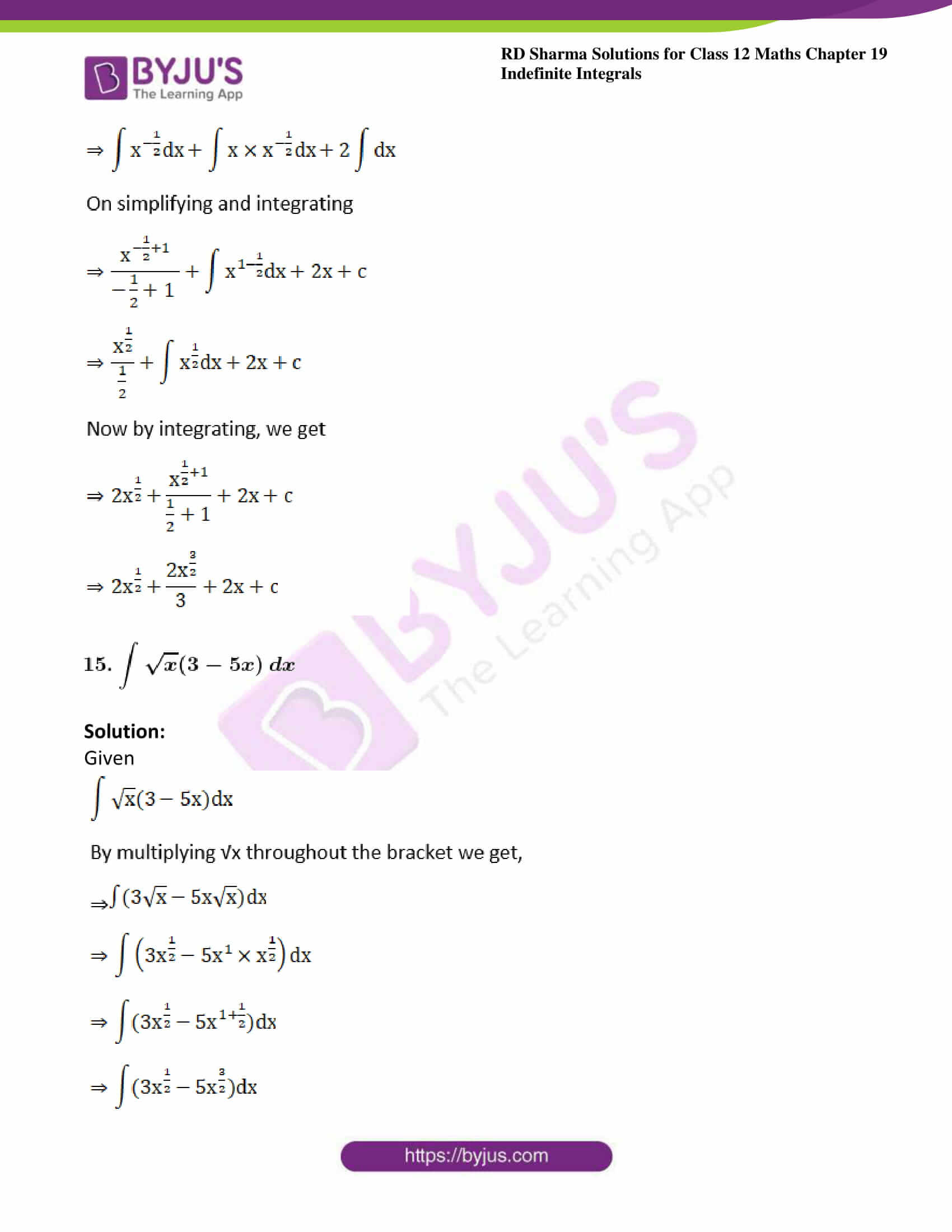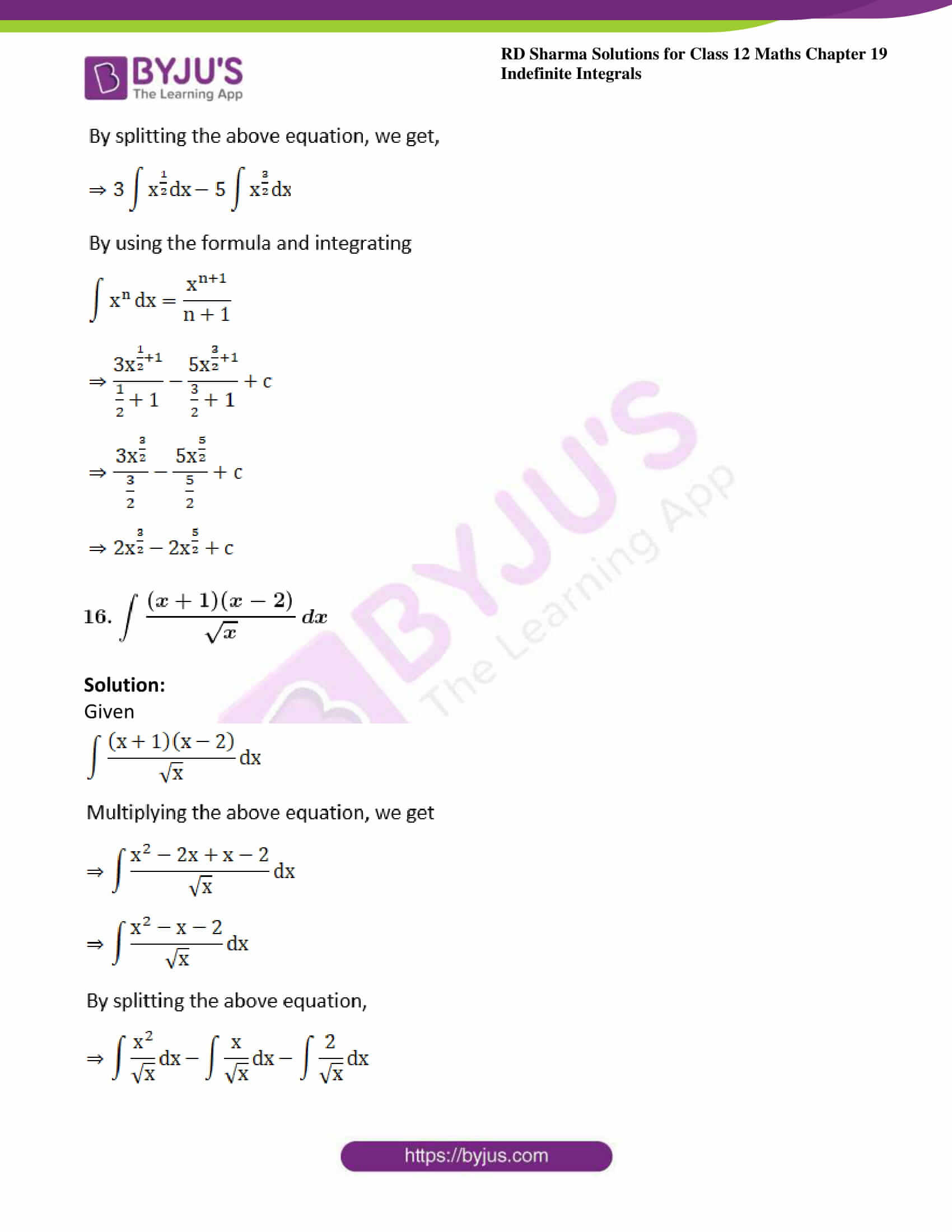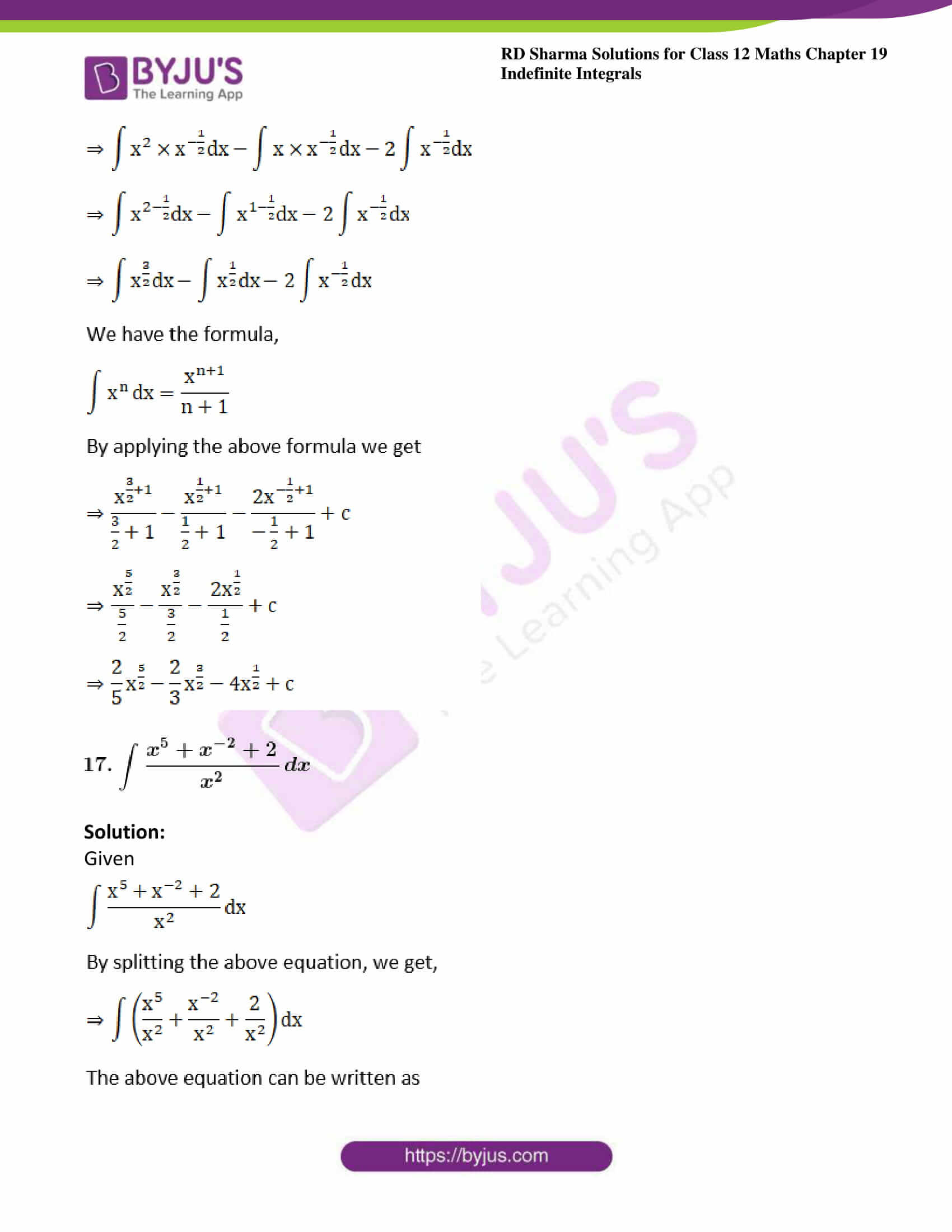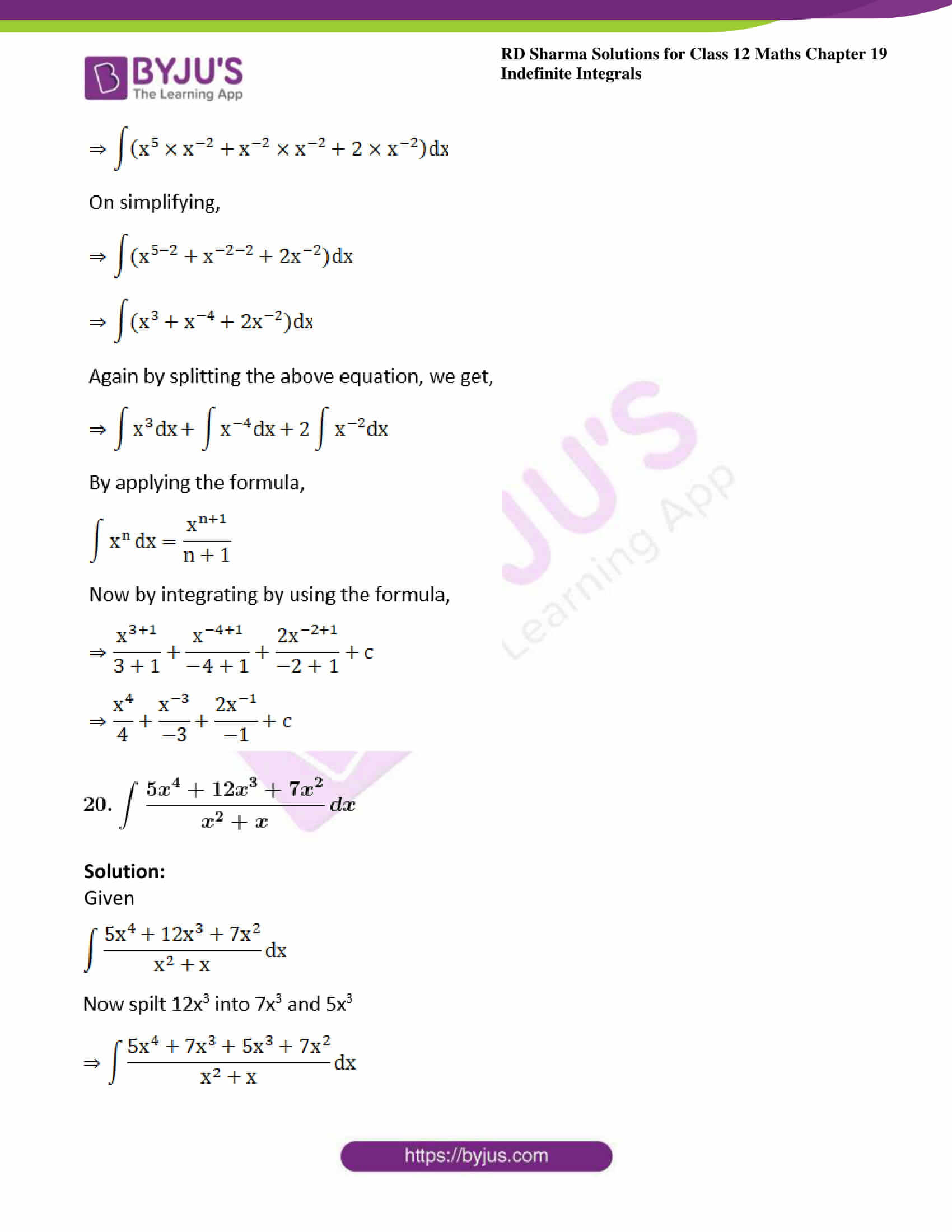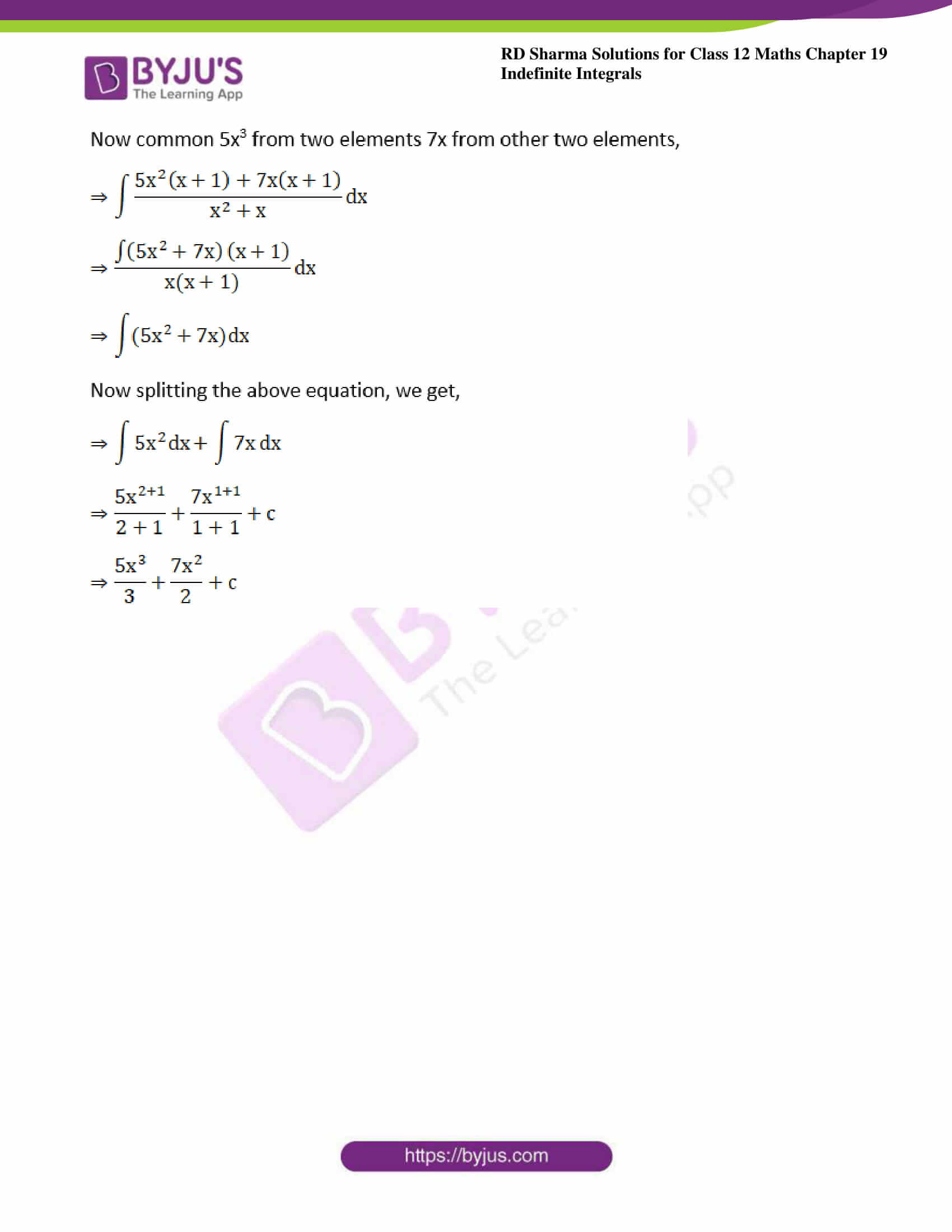### Access answers to Maths RD Sharma Solutions For Class 12 Chapter 19 – Indefinite Integrals Exercise 19.2

Evaluate the following integrals (1 – 44):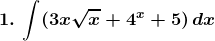Solution: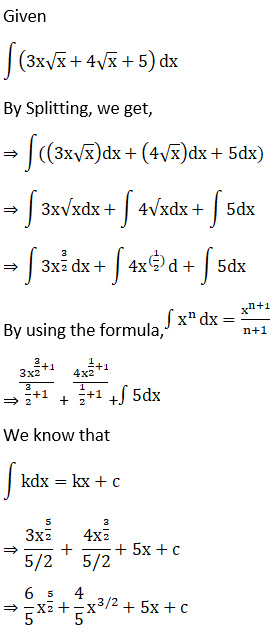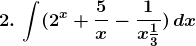Solution:

Given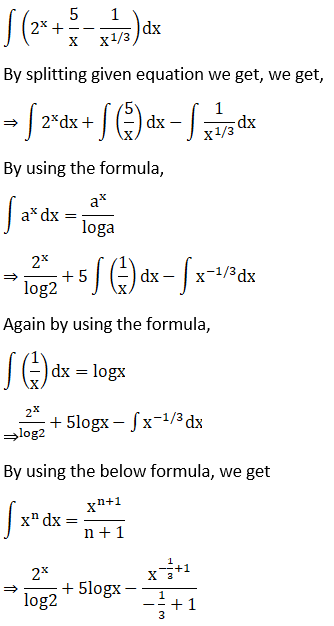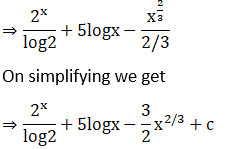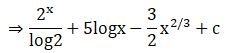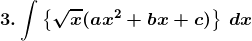Solution:

Given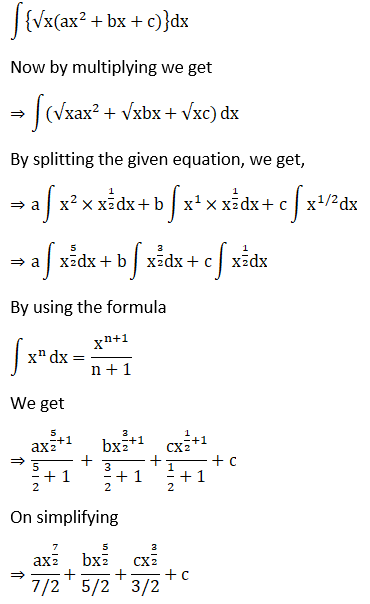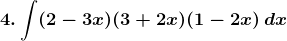Solution:

Given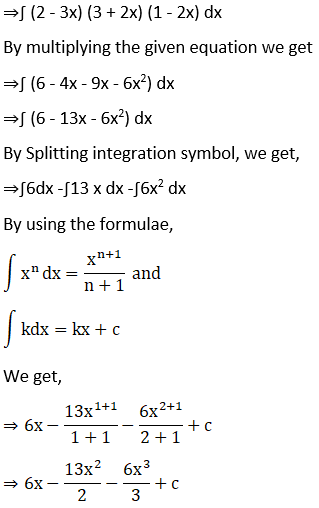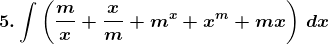Solution:

Given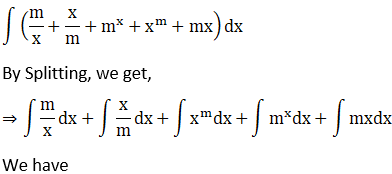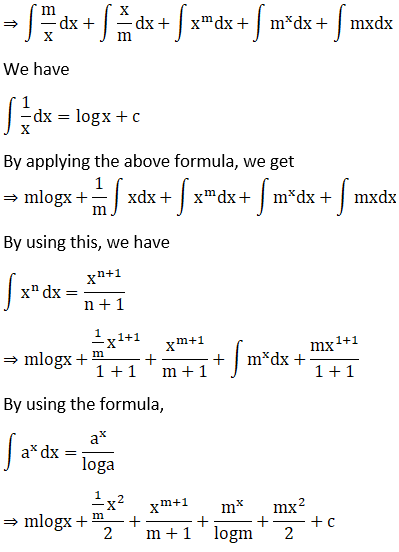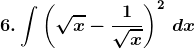Solution: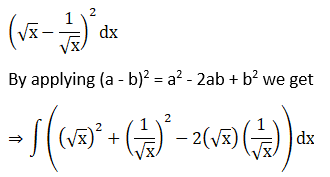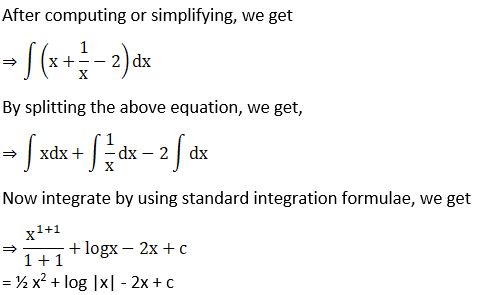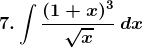Solution:

Given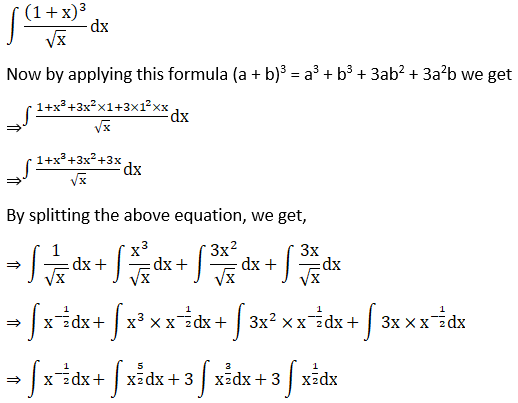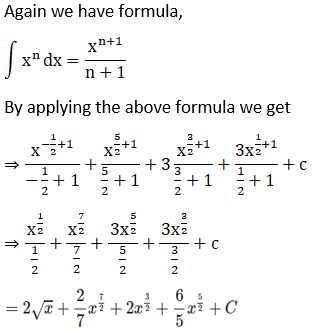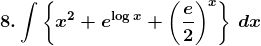Solution:

Given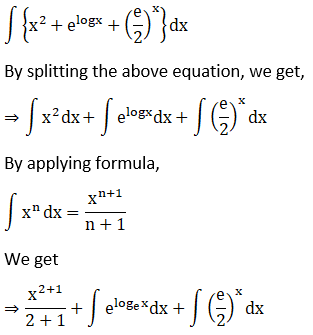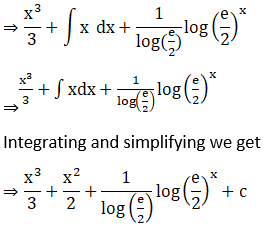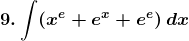Solution: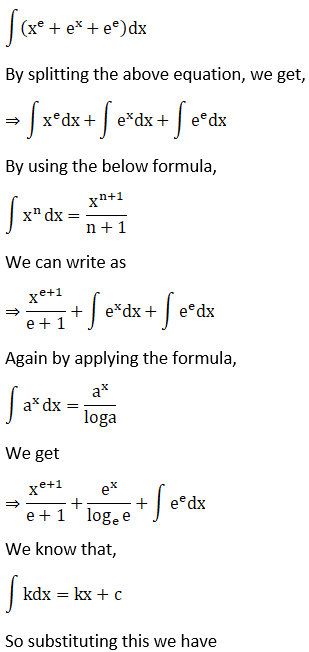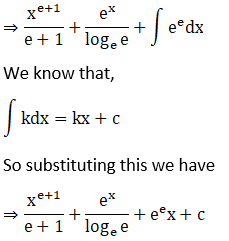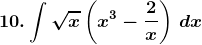Solution:

Given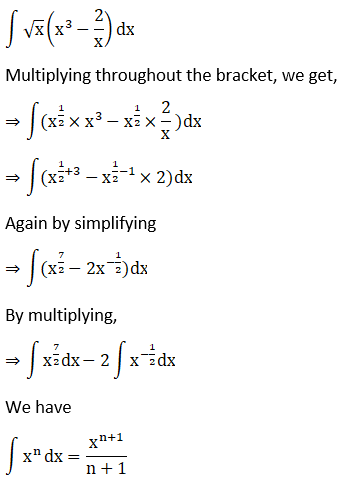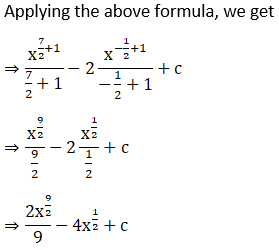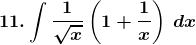Solution:

Given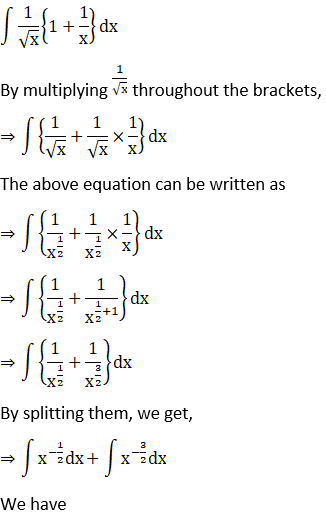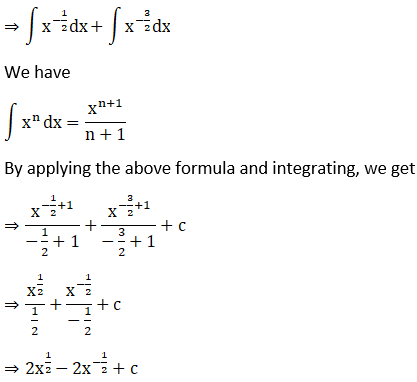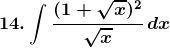Solution: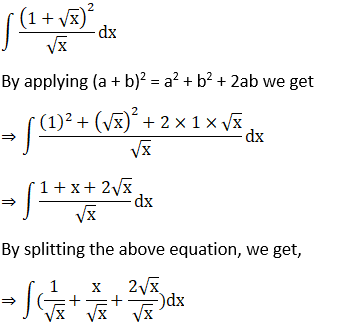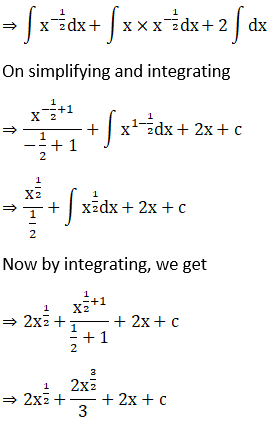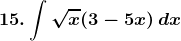Solution:

Given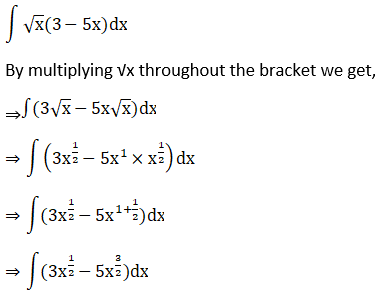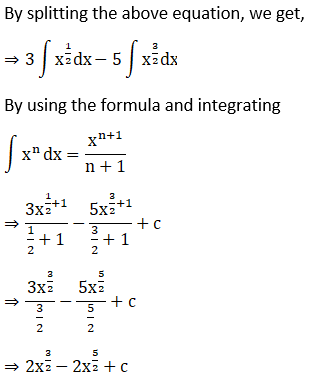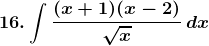Solution:

Given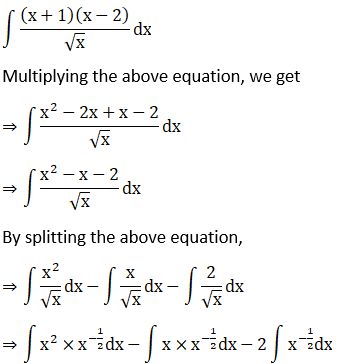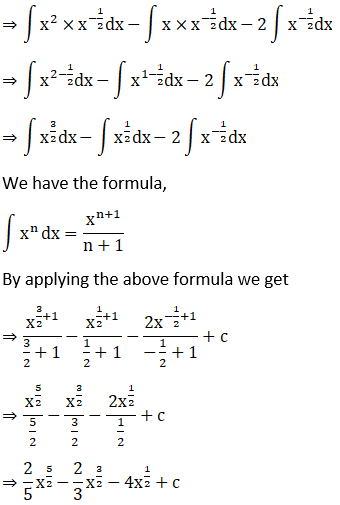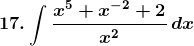Solution:

Given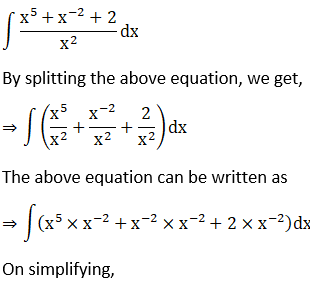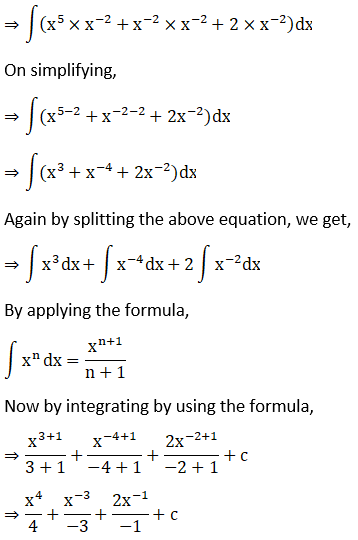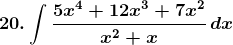Solution:

Given